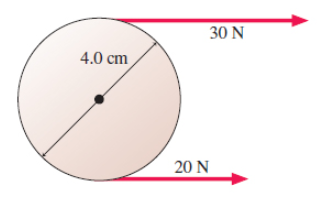# Problem: In the figure (Figure 1), what is the net torque about the axle? Express your answer to two significant figures and include the appropriate units.

###### FREE Expert Solution

Let clockwise be positive and counter-clockwise negative.

87% (311 ratings)###### Problem Details

In the figure (Figure 1), what is the net torque about the axle? Express your answer to two significant figures and include the appropriate units.